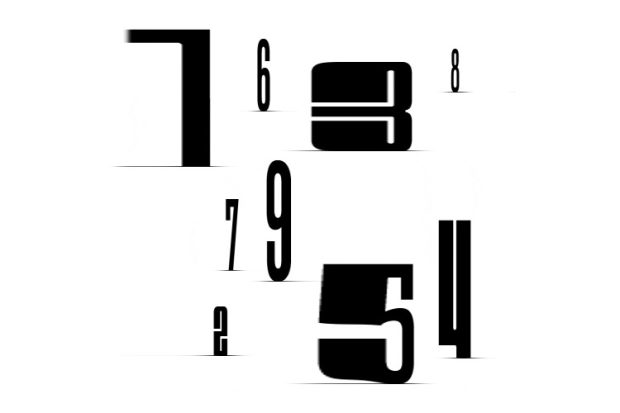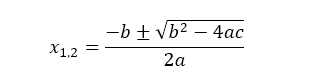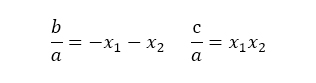# Group Theory

## Mathematician Gareth Jones on abelian and non-abelian groups, the symmetry of geometric objects and what are the principles a group must satisfy

### videos | July 9, 2020

Group theory is a branch of algebra, and it’s sometimes described as the mathematical theory of symmetry. What I’d like to do is explain how it arises, how it can be applied and give you some other history.

The definition of a group is rather technical. It involves a couple of axioms, which I’m not going to write down precisely; what I will do is describe three basic principles which a group must satisfy, and I’ll do it by giving you a couple of examples. The first area in which we find examples of groups is in the number system. Let’s take the integers as an example. Two integers can be combined by adding them to produce a third integer. There is an integer which does nothing when you add it: it’s called zero. A plus zero is the same as A. That is called an identity element in the group. Given any integer a, there is an opposite integer which cancels it out: it’s called –a. If I add a and –a, I get zero.

Other number systems have the same properties. For instance, it works with real numbers; you can add polynomials, and they satisfy the same rules; you can add vectors you can add matrices, and they all satisfy those three basic rules. They are the rules which characterize a group: you have a set, you have a way of combining two elements in the set to produce a third, you have an identity element which does nothing when you combine it with something else, and you have inverses, every element has an inverse which cancels it out.Mathematician James Maynard on the Riemann Hypothesis, Euclid's proof, and the problems of Paul Erdős
I’ve given you addition as an example, but the same sort of thing happens with multiplication. Let’s take the set of nonzero real numbers: I’ll explain why we have to exclude zero in a moment. If I multiply two of them, I’ll get another nonzero real number. There is a nonzero real number which does nothing when you multiply by it: it’s called 1. A ∙ 1 is the same as A. Every nonzero real number has an inverse, namely 1/a, and that is why we need to exclude zero: zero does not have an inverse 1/0; it doesn’t mean anything. So, nonzero real numbers form a group under multiplication, and there are other examples: nonzero complex numbers and nonzero rational numbers. You can even form a group out of matrices, providing you exclude certain matrices, those with the determinant 0, because they don’t have inverses.

So that’s one area where we find examples of groups. Another area is in studying the symmetry of geometric objects like regular solids. I have two examples here: a cube and an icosahedron with 20 triangular faces. Each of these geometric objects exhibits some form of symmetry: I can rotate them until they occupy the same part of space. For instance, I can rotate this cube through 90 degrees, and it occupies the same space as before. I can rotate it in various directions. It’s an easy exercise to count the number of rotations: there are 24 of them altogether, including the identity rotation, where I don’t move it at all. The point is that I can combine any two rotations, and I will get a third rotation; there is a rotation that does nothing, and any rotation can be reversed, so any rotation has an opposite rotation. So these rotations form a group: in the case of the cube, there are 24 of them; in the case of the icosahedron, there are 60 of them, and again, it’s an easy exercise to count them.

If we go back to the cube, let’s now think of it as a Rubik’s Cube and allow ourselves to do the usual twists. Any twist can be undone; I can reverse it, and there is a twist through zero degrees, the twist that does nothing. So, these moves of the Rubik’s Cube form a group. The group is much larger: the number of elements is about 4 ∙ 1019. If you were an expert on the Rubik’s Cube and you could move it around really fast, you could go through all of these different elements of the group at 100 moves per second; if you started at the time of the Big Bang when the universe started, you would just about have come towards the end by now: that is how big this group is. So, a really simple geometric object like this can embody some really complicated mathematics.

Let’s jump to an area which exhibits symmetry. We find symmetry in many areas of science as well: crystallography, for example, quantum theory, particle physics, etc. They are all very highly dependent on group theory principles because they are built upon symmetry, but that is not historically how group theory arose. History got it wrong in a sense. Group theory should have arisen out of early studies of the number system or of symmetric objects, but it didn’t: it arose about 200 years ago out of a study of polynomials. At school, we are taught how to solve a quadratic equation, one involving x2 but no higher powers of x, ax2 + bx + c = 0, where a, b and c are constants and x is the variable, and the problem is to find x.

The solution is:It doesn’t matter what is actually in that formula; you don’t need to remember it, but I want you to notice that it includes the four operations of arithmetic, namely addition, subtraction, multiplication and division, together with one more, namely that square root sign.

In the Renaissance, Italian mathematicians were trying to find analogous formulae for the roots of cubic polynomials and higher-degree polynomials. They succeeded with cubic, and they succeeded with fourth-degree polynomials; they found similar formulae: they’re more complicated, they involve the four operations of arithmetic and taking nth roots for various values of n. This process is called solving the polynomial by radicals. Radicals mean nth roots. So, they were able to solve polynomials of degrees up to and including four by these operations of arithmetic.Mathematician Gareth Jones on Gödel's incompleteness theorem, the halting problem and why the subsets of the natural numbers are uncountably infinite
The obvious question then arose: can we do this for the fifth-degree polynomial, what’s called the quintic polynomial? For 200 years, mathematicians tried, and they all failed. Towards the end of the 18th century, they started to suspect that it was impossible. In 1799, an Italian mathematician called Ruffini published a proof that it was impossible. His proof was incomplete, and no one really understood it, but in 1824, a Norwegian mathematician called Abel published a complete proof that there is no analogous formula for the fifth-degree polynomial. A couple of years later, a French mathematician, a young man called Galois, developed the general theory, which allows us to determine exactly which polynomials can and which polynomials cannot be solved by radicals.

How did they do this? They used the symmetry properties of polynomials. Let me go back to the example of the quadratic formula. You can express the ratios of the coefficients a, b and c in terms of the roots, and in each case, on the right-hand side, you have a symmetric function of the roots:If I commute x1 and x2, if I make them change places, the right-hand sides are not changed. This happens for the quadratic polynomials, and there are similar formulae for all polynomials of any degree. You have what is called the symmetric functions of the roots appearing on the right-hand side, and this means that polynomials have symmetries just like the regular solids I showed you a few minutes ago: they have groups of symmetries, and one can exploit the algebraic properties of these groups to study the polynomials.

In the case of a polynomial of degree n, the group that arises is the group of all permutations of the roots. This is called the symmetric group of degree n. In the case of the fifth-degree polynomial, the number of elements is 120, so it’s a reasonably complicated group but not enormously complicated. The polynomials of degrees 2, 3 and 4, these symmetric groups can be broken down into easy pieces, what we call abelian groups. These are groups in which a composed with b is the same as b composed with a. This happens in all the number systems: a + b is the same as b + a, for example.

It doesn’t happen in the case of symmetries. Let me illustrate this with a Rubik’s cube. Suppose that a is rotation forward by 90 degrees, and b is rotation to my left by 90 degrees. If I do a first and then b, the red tiles are in front. If I undo it, we’re back to where we started. Now I will do b first, and then I will do a, and the blue tiles are in front. So, a followed by b is not the same as b followed by a.

We notice this in everyday life: most people when they get dressed put their socks on before their shoes. There’s no law that says you have to do that: you could put your shoes on first and then put your socks on but it would be rather strange if you did that. So there’s an example of a non-abelian group: shoes and socks for the non-abelian group.

These polynomials of degree at most 4 have the symmetric group of degree at most four as their symmetry group, and that symmetry group can be broken down into easy abelian pieces. These abelian pieces tell us how to write down the formula involving nth roots. When you try this with degree 5, this group of order 120, you can reduce it to a group of half the size, namely 60, but then you cannot reduce that group any further; you cannot break it down into easy abelian pieces. It’s what’s called a simple group: that doesn’t mean any easy group; it means simple in the sense that it cannot be reduced any further. In fact, we’ve seen that group already: it’s the same structurally as the rotation group of the icosahedron. You can see this from the colouring of the icosahedron: there are five colours used there, and the rotations permute the colours, and the permutations of the colours are exactly the same as those appearing in this group of order 120. This connection between the quintic polynomial and the icosahedron is so important and so interesting that Felix Klein wrote a whole book called ‘Lectures on the icosahedron’.

So, that is where group theory comes from. It’s still a living subject; Abel and Galois died young. Unfortunately, they died in their twenties, but they left us with a very important and very interesting theory. I’ve spent half a century working on it, and I’m still learning something.Emeritus Professor of Mathematics, University of Southampton
Did you like it? Share it with your friends!
Published items
0779
To be published soon
+92

#### Most viewed

• 1Peer Fischer
• 2Diana Omigie
• 3Patrick Haggard
• 4Ugo Moschella
• 5•